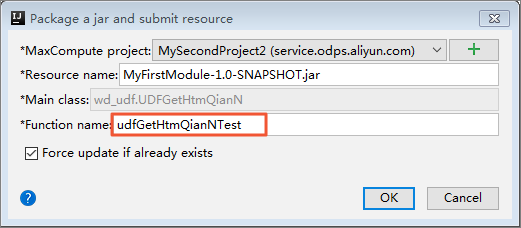## 获取URL中指定位置的字符UDF说明

`String UDFGetHtmQianN(String url, Long n)`
• 函数名：`UDFGetHtmQianN`
• 功能：获取URL中指定位置的字符。
• 参数说明：
• `url`：STRING类型URL。
• `n`：LONG类型长度值。

## UDF使用示例

• 注册函数
UDFGetHtmQianN.java测试通过后，将其注册为函数使用。说明 一个UDF从发布到服务端供生产使用，需要经过打包、上传、注册这三个步骤。您可以使用一键发布功能一次性完成这些步骤（Studio会依次执行`mvn clean package`、上传Jar和注册UDF这三个步骤）。详情请参见打包、上传和注册
• 使用示例
成功注册UDF后，执行以下命令：
• 示例一
``select udfGetHtmQianNTest("http://www.taobao.com", 1) from dual;``
运行结果如下。
``````+-----+
| _c0 |
+-----+
|     |
+-----+``````
说明 这里的返回结果不为NULL。
• 示例二
``select udfGetHtmQianNTest("http://www.taobao.com/a.htm", 1) from dual;``
运行结果如下。
``````+-----+
| _c0 |
+-----+
| a   |
+-----+``````
• 示例三
``select udfGetHtmQianNTest("http://www.taobao.com/a-b-c-d.htm", 3) from dual;``
运行结果如下。
``````+-----+
| _c0 |
+-----+
| b   |
+-----+``````

## UDF代码示例

• UDF实现示例
``````package com.aliyun.odps.examples.udf// package名称，可以根据您的情况定义。
import com.aliyun.odps.udf.UDF;
public  class  UDFGetHtmQianN  extends  UDF  {
public  String  evaluate(String  url,  Long  n)  {
try  {
// 是取url字符串中第一次出现.htm时.的索引位置，数值类型为int。
int  index  =  url.indexOf(".htm");
if  (index  <  0)  {
return  "";
}
// 从0开始数，其中不包括index位置的字符。
String  a  =  url.substring(0, index);
// 返回指定字符/在此字符串中最后一次出现处的索引。
index  =  a.lastIndexOf("/");
// 取得的字符串长度为：a.length() - (index  +  1)
String  b  =  a.substring(index  +  1, a.length());
// 用"-"拆分字符串b，获取字符串数组。
String[]  c  =  b.split("-");
// 此处c.length>=n时，才有返回值。
if  (c.length  <  n)  {
return  "";
}
if  (n  ==  0)  {
return  "";
}
// 返回字符串数组中指定下标的字符。
String  d  =  c[c.length  -  n.intValue()];
return  d;
}  catch  (Exception  e)  {
return  "err";
}
}
}``````
• UDF单元测试
``````package com.aliyun.odps.examples.udf// package名称，可以根据您的情况定义。
import org.junit.Test;
import static org.junit.Assert.assertEquals;
public  class  TestUDFGetHtmQianN  {
private  UDFGetHtmQianN  udf  =  new  UDFGetHtmQianN();

@Test
public  void  test_url(){
Long  n  =  1L;
assertEquals("err",  udf.evaluate(null,  n));
//  原来抛异常，现返回null。
assertEquals("",  udf.evaluate("",  n));

assertEquals("",  udf.evaluate("http://www.taobao.com",  n));
assertEquals("a",  udf.evaluate("http://www.taobao.com/a.htm",  n));
assertEquals("b",  udf.evaluate("http://www.taobao.com/a-b.htm",  n));
}

@Test
public  void  test_index(){
String  url  =  "http://www.taobao.com/a-b-c.htm";
assertEquals("err",  udf.evaluate(url,  null));
//  原来抛异常，现返回null。
assertEquals("err",  udf.evaluate(url,  -1L));
assertEquals("",  udf.evaluate(url,  0L));

assertEquals("c",  udf.evaluate(url,  1L));
//  原来返回-,  现返回null。
assertEquals("",  udf.evaluate(url,  100L));
}
}``````